# NUMERICAL EVALUATION OF THE EFFECT OF TYPE AND SHAPE OF PERFORATIONS ON THE BUCKLING OF THIN STEEL PLATES BY MEANS OF THE CONSTRUCTAL DESIGN METHOD

NUMERICAL EVALUATION OF THE EFFECT OF TYPE AND SHAPE OF PERFORATIONS ON THE BUCKLING OF THIN STEEL PLATES BY MEANS OF THE CONSTRUCTAL DESIGN METHOD

Giulio Lorenzini Daniel Helbig Caio Cesar Cardoso da Silva Mauro de Vasconcellos Real Elizaldo Domingues dos Santos Liércio André Isoldi Luiz Alberto Oliveira Rocha

Department of Industrial Engineering, University of Parma, Parco Area delle Scienze, 181/A, Parma, 43124, Italy

Programa de Pós-Graduação em Engenharia Mecânica (PROMEC), Universidade Federal do Rio Grande do Sul (UFRGS), Sarmento Leite St. nº 425, Porto Alegre, 90050-170, Brazil

Programa de Pós-Graduação em Engenharia Oceânica (PPGEO), Universidade Federal do Rio Grande (FURG), Itália Ave. km 8, Rio Grande, 96203-900, Brazil

Corresponding Author Email:
giulio.lorenzini@unipr.it
Page:
S9-S20
|
DOI:
https://dx.doi.org/10.18280/ijht.34S102
|
Accepted:
|
Published:
31 January 2016
| Citation

OPEN ACCESS

Abstract:

Thin steel plates - with or without cutouts - are structural components largely used in several engineering applications as buildings, bridges, ships, airplanes and automobiles. However, if an axial compressive load is imposed to these panels an undesired instability phenomenon can occur: buckling. At a certain load magnitude the limit stress is reached and the plate suffers lateral displacements (out of plane) indicating the buckling occurrence. In plates an elastic buckling or an elasto-plastic buckling can occur, depending on dimensional, constructive or operational aspects. Therefore, in the present work, the Constructal Design method was adopted to investigate the influence of the type and shape of the cutout in the plate buckling. To do so, by means the Finite Element Method (FEM), computational models were developed to simulate the elastic (linear) and elasto-plastic (nonlinear) plate buckling. Square and rectangular thin steel plates, simply supported in its four edges, with a centered cutout, were analyzed, being the objective function to maximize the buckling limit stress, avoiding the plate buckling occurrence. The square and rectangular plates have a ratio H/L (ratio between height and length of the plate) of 0.5 and 1.0, respectively. A value of 0.2 for the cutout volume fraction (ratio between the cutout volume and the total plate volume) was adopted for different types of cutout: diamond, longitudinal hexagonal, transversal hexagonal, elliptical, and rectangular. The cutout shape variations were produced by the H0/L0 degree of freedom (which relates the characteristic dimensions of the cutout). The results showed that the cutout shape variation has a fundamental influence in the plate buckling behavior, determining if the buckling is elastic or elasto-plastic, allowing the definition of a buckling stress limit curve for each studied cutout type. In addition, it was observed that the Constructal Design method conduct to the definition of optimal geometries, reaching buckling stress limit improvements around 100%.

Keywords:

Constructal design, Thin steel plate with cutout, Linear elastic buckling, Nonlinear elasto-plastic buckling, computational modeling.

1. Introduction

Thin steel plate elements constitute very important structural components in many structures, such as ship decks and hulls, dock gates, plate and box girders of bridges, oﬀshore structures, and structures used in aerospace industries. In many cases, these plates are subjected to axial compressive forces, which make them prone to instability or buckling. If the plate is slender, the buckling is elastic. However, if the plate is sturdy, it buckles in the plastic range causing the so-called inelastic (or elasto-plastic) buckling. It is very likely in many cases to have holes in the plate elements for inspection, maintenance, and service purposes, and the size of these holes could be signiﬁcant. In such cases, the presence of these holes redistributes the membrane stresses in the plate and may cause signiﬁcant reduction in its strength in addition to changing its buckling characteristics, El-Sawy et al. .

The buckling of a plate involves two planes, and two boundary conditions on each edge of the plate. The basic difference between a column and a plate lies in the buckling characteristics. A column, once it buckles, cannot resist any additional axial load. Thus, the critical load of a column is also its failure load. On the other hand, a plate, since it is invariably supported at the edges (e.g., interconnection between two structural plates, and web connected to flanges), continues to resist the additional axial load even after the primary buckling load. Thus, for a plate, the post-buckling load is much higher than the elastic buckling load. When designing structural members, this fact is largely exploited to minimize the weight of the structure, Iyengar .

Moreover, in several practical applications it is necessary to provide cutouts in plate structures to allow access for services or inspection and even aesthetics purposes, as well as to reduce the structure self-weight. The presence of a hole in a plate panel changes the stress distribution within the member, alters its elastic buckling and post-buckling characteristics and generally reduces its ultimate load carrying capacity. The performance of a plate containing an opening is influenced by the nature of the applied stress (e.g. compressive, tensile, shear, etc.), besides the shape, size and location of the hole, Narayanan .

Among the elastic buckling studies category, Sharkeley & Brown , studied the effect of eccentricity on square plates with square holes. They concluded that the center of a small square hole should be located away from the center of the structural element, but the center of a large square hole should be located on the center of the structural element. El-Sawy & Nazmy , provided a comprehensive discussion on the elastic buckling of thin rectangular perforated plates for various hole shapes, sizes, and locations. El-Sawy & Martini  used the finite element method to determine the elastic buckling stresses of biaxial loaded perforated rectangular plates with longitudinal axis located circular holes. Alternatively, Moen & Schafer  developed, validated and summarized analytical expressions for estimating the influence of single or multiple holes on the elastic buckling critical stress of plates in bending or compression. In Rocha et al. [8, 9] and Isoldi et al. , the Constructal Design method was employed to determine the best shape and size of centered cutout in a plate, aiming to maximize the critical buckling load.

Considering the above, it appears of fundamental importance in the structural engineering, the study and understanding of the mechanical behavior of perforated steel plates submitted buckling, especially if the goal is to improve the performance of these structural elements. Therefore, the main objective of this work is numerically investigate the influence of the type and shape of the hole in the behavior of buckling perforated steel plates, in order to improve its mechanical behavior. The Constructal Design method is used in order to guarantee an adequate and consistent comparison among the studied cases. The objective function is to maximize the compressive stress, avoiding the plate buckling occurrence. To do so, it was considered a hole volume fraction (ratio between the hole and the volume of the total volume of the plate) of 0.20, for different cutout types: diamond, longitudinal hexagonal, transversal hexagonal, elliptical, and rectangular. The shape of these perforations can vary by means the ratio H0/L0, which relates the characteristics dimensions of each hole. Besides, two ratios between the plate height (H) and the plate length (L) were studied: H/L = 0.5 and H/L = 1.0: emphasizing that the total volume of the plate was kept constant, with a plate thickness (t) of 10 mm. In all studied cases, the plate is simply supported in its four edges and has a centered perforation. A constraint which limit a minimal distance of 100 mm from the plate edges until the hole edges is also employed.

2. Buckling and Postbuckling of Plates

In the late 1800s, Bryan successfully formulated and solved the problem of buckling for a linearly elastic and simply supported uniaxial compressed plate. Approximately 33 years later, Bleich made efforts to extend linear elastic plate buckling theory to the solution of problems of plates buckling above the proportional limit. He demonstrated that critical stresses for plates buckling between the proportional limit and the yield stress could be approximated using linear elastic theory with Young’s modulus, E, replaced by a reduced value equal to $\sqrt{E_{t} E}$, where Et is the tangent modulus. Timoshenko agreed with this observation and further concluded that plate buckling stresses cannot exceed the yield stress, Dawe et al. .

For a common man, the word buckling means sudden catastrophic failure of a structure involving large deformations. But in engineering parlance, the buckling is a phenomenon that generally occurs well before deformations are very large. When a slender structure is loaded in compression, for small loads it deforms with hardly any noticeable change in geometry and load-carrying capacity. On reaching a critical load value, the structure suddenly experiences a large deformation and it may lose its ability to carry the load further. At this stage, the structure is considered to have buckled, Raviprakash .

The transition of the plate from the stable state of equilibrium to the unstable one, when submitted a compressive load, is referred to as buckling or structural instability. The smallest value of the load producing buckling is called the critical or buckling load. The importance of buckling is the initiation of a deflection pattern, which if the loads are further increased above their critical values, rapidly leads to very large lateral deflections. Consequently, it leads to large bending stresses, and eventually to complete failure of the plate. The linear buckling analysis of plates based on these assumptions makes it possible to determine accurately the critical loads (Pcr), which are of practical importance in the stability analysis of thin plates. However, this analysis gives no way of describing the behavior of plates after buckling, which is also of considerable interest. The post-buckling analysis of plates is usually difﬁcult because it is basically a nonlinear problem, Ventsel .

## 1.png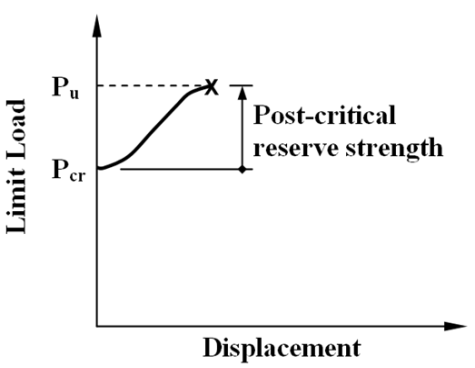Figure 1. Load/displacement diagram in the post-critical range

This capacity to carry additional load after elastic buckling is due to the formation of a membrane that stabilizes the buckle through a transverse tension band. When the central part of the plate buckles, it loses the major part of its stiffness, and then the load is forced to be “linked’’ around this weakened zone into the stiffer parts on either side. Additionally, due to this redistribution a transverse membrane in tension is formed and anchored, as can be seen by the load paths in Fig. 2, Åkesson .

## 2.png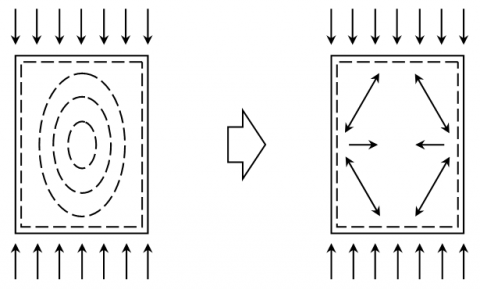Figure 2. The redistribution of the transfer of load in the ultimate limit state

The relative magnitude of the post-buckling strength to the buckling load depends on various parameters such as dimensional properties, boundary conditions, types of loading, and the ratio of buckling stress to yield stress, Yoo & Lee . There is an analytical solution for the problem of the elastic buckling of a simply supported solid plate of length L, width H, thickness t, and subjected to a distributed uniaxial load P as it is shown in Fig. 3.

## 3.png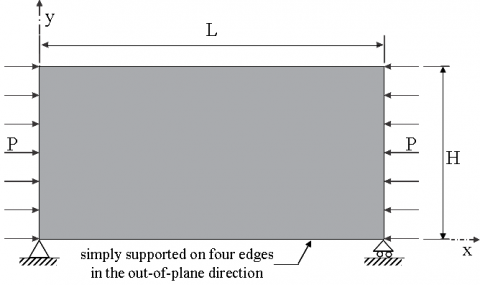Figure 3. The solid plate subject to uniaxial compressive load

The critical load per unit length for elastic buckling can be written according to, Vinson  as:

$P_{c r}=k \frac{\pi^{2} D}{H^{2}}$(1)

Where π is the mathematical constant; k is the function of aspect radio H/L and wavelength parameter m, given by:

$k=\left(m \frac{H}{L}+\frac{1}{m} \frac{L}{H}\right)^{2}$(2)

and D is the plate bending stiffness, defined as:

$D=\frac{E t^{3}}{12\left(1-v^{2}\right)}$(3)

Where E is the Young’s Modulus and v is the Poisson’s ratio of the plate material.

The optimum value of m that gives the lowest σcr depends on the aspect ratio H/L. For example, the optimum m is 1.0 for a square plate (H/L = 1.0) while it is 2.0 for a plate of (H/L = 2.0). For a plate with a large aspect ratio, k = 4.0 serves as a good approximation. Since the aspect ratio of a component of a steel structural member such as a web plate is large in general, we can often assume k is simply equal to 4.0, Yamaguchi .

In turn, the stress at which elastic buckling occurs, σcr, is defined by the average stress that is equal to the uniformly applied compressive load, Pcr, divided by the thickness of the plate, t. This stress is called elastic buckling stress, and it is given by:

$\sigma_{c r}=k \frac{\pi^{2} E}{12\left(1-v^{2}\right)}\left(\frac{t}{H}\right)^{2}$(4)

3. Computational Models

There are many practical engineering problems for which exact solutions cannot be obtained. This inability to obtain an exact solution may be attributed to either the complex nature of governing differential equations or the difficulties that arise from dealing with the boundary and initial conditions. To deal with such problems, one may resort to numerical approximations. In contrast to analytical solutions, which show the behavior of a system at any point within the system, numerical solutions approximate the solutions only at discrete points, called nodes. The first step of any numerical procedure is the discretization. This process divides the medium of interest into a number of small subregions and nodes. There are two common classes of numerical methods: finite difference methods (FDM) and finite element methods (FEM). With FDM, the differential equation is written for each node, and the derivatives are replaced by difference equations. This approach results in a set of simultaneous linear equations. Although finite difference methods are easy to understand and employ in simple problems, they become difficult to apply to problems with complex geometries or complex boundary conditions. This situation is also true for problems with nonisotropic material properties. In contrast, the FEM uses integral formulations rather than difference equations to create a system of algebraic equations. Moreover, an approximate continuous function is assumed to represent the solution for each element. The complete solution is then generated by connecting or assembling the individual solutions, allowing for continuity at the interelemental boundaries, Moaveni .

In this context, the ANSYS® software, which is based on the FEM, was used to solve elastic and elasto-plastic plate buckling problems. A set of interpolation functions is used to define uniquely the state of displacement within each element in terms of its nodal displacements. The state of strain within the element is uniquely defined by the strain-displacement relationship. The state of stress throughout the element is determined by the material stress-strain law. By applying the Virtual Work Principle, the nodal forces corresponding to a displacement field in the element are determined. These nodal forces are related to the nodal displacements through the element stiffness matrix. Thus, the conditions of overall equilibrium have already been satisfied within the element. Now, all that is necessary is to establish equilibrium conditions at the nodes of the structure. The resulting linear equation system will contain the displacements as unknowns. Once these equations have been solved the structural problem is determined. The internal forces in elements, or the stresses, can easily be found by using the strain-displacement relationship and the material stress-strain law, Real & Isoldi . The analytical solutions are only available for problems involving very simple geometry, loading and boundary conditions. Computer modeling can be used to search approximate solution to solve more complex problems, Helbig et al. .

In the present work the 8-Node Structural Shell finite element so-called SHELL93 was used (Fig. 4). This element is particularly well suited to model curved shells. The element has six degrees of freedom at each node: translations in the nodal x, y and z directions and rotations about the nodal x, y and z axes. The deformation shapes are quadratic in both in-plane directions. The element has plasticity, stress stiffening, large deflection, and large strain capabilities, Ansys® .

## 4.png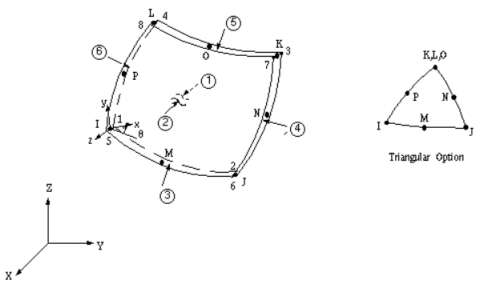Figure 4. SHELL93 finite element 

3.1 Elastic buckling

Eigenvalue linear buckling analysis is generally used to estimate the critical buckling load of ideal structures Vinson . This numerical procedure is used for calculating the theoretical buckling load of a linear elastic structure. Since it assumes the structure exhibits linearly elastic behavior, the predicted buckling loads are overestimated. So, if the component is expected to exhibit structural instability, the search for the load that causes structural bifurcation is referred to as a buckling load analysis. Because the buckling load is not known a priori, the finite element equilibrium equations for this type of analysis involve the solution of homogeneous algebraic equations whose lowest eigenvalue corresponds to the buckling load, and the associated eigenvector represents the primary buckling mode, Madenci & Guven .

The strain formulation used in the analysis includes both the linear and nonlinear terms. Thus, the total stiffness matrix, [K], is obtained by summing the conventional stiffness matrix for small deformation, [KE], with another matrix, [KG], which is the so-called geometrical stiffness matrix, Przemieniecki . The matrix [KG] depends not only on the geometry but also on the initial internal forces (stresses) existing at the start of the loading step, {P0}. Therefore the total stiffness matrix of the plate with load level {P0} can be written as:

$[K]=\left[K_{E}\right]+\left[K_{G}\right]$(5)

When the load reaches the level of {P} = λ{P0}, where l is a scalar, the stiffness matrix can be defined as:

$[K]=\left[K_{E}\right]+\lambda\left[K_{G}\right]$(6)

Now, the governing equilibrium equations for the plate behavior can be written as:

$\left[\left[K_{E}\right]+\lambda\left[K_{G}\right]\right]\{U\}=\lambda\left\{P_{0}\right\}$(7)

where $\{U\}$ is the total displacement vector that may therefore be determined from:

$\{U\}=\left[\left[K_{E}\right]+\lambda\left[K_{G}\right]\right]^{-1} \lambda\left\{P_{0}\right\}$(8)

At buckling, the plate exhibits a large increase in its displacements with no increase in the load. From the mathematical definition of the matrix inverse as the adjoint matrix divided by the determinant of the coefficients it is possible to note that the displacements $\{U\}$ tend to infinity when:

$\operatorname{det}\left[\left[K_{E}\right]+\lambda\left[K_{G}\right]\right]=0$(9)

Equation (9) represents an eigenvalue problem, which when solved provides the lowest eigenvalue, λ1 that corresponds to the critical load level {Pcr} = λ1{P0} at which buckling occurs. In addition, the associated scaled displacement vector $\{U\}$defines the mode shape at buckling. In the finite element program ANSYS®, the eigenvalue problem is solved by using the Lanczos numerical method, Ansys® .

3.2 Elasto-plastic buckling

Nonlinear or collapse buckling analysis is a more accurate approach since this finite element analysis has capability of analyzing the actual structures with imperfections. This approach is highly recommended for design or evaluation of actual structures. This technique employs a non-linear structural analysis with gradually increasing loads to seek the load level at which the structure become unstable. Using this technique, features such as initial imperfections, plastic behavior etc., can be included in the model. In this analysis, both geometrical and material nonlinearities are considered. A shell is said to behave nonlinearly if the deflection at any point is not proportional to the magnitude of the applied load, Budiansky . The geometric nonlinearity is the result of nonlinear strain-displacement relations, and the material nonlinearity is the result of nonlinear stress-strain relations. The material non-linearities can also be defined with different work hardening behaviors, Avner .

To do so, the plate material was assumed to be linear elastic–perfectly plastic (i.e., with no strain hardening) which is the most critical case for the steel material. An initial imperfect geometry for the plate that follows the first buckling mode of its elastic eigenvalue pre-analysis is assumed. The maximum value of the imperfection is chosen to be H/2000, El-Sawy et al. , being H the plate width (see Fig. 3).

To find out the plate ultimate load, a reference load given by Py = σy.t, where σy is the material yielding strength, was applied in little increments in the plate edge parallel to the y axis. For each load increment the standard Newton-Raphson method was applied to determine the displacements that correspond to the equilibrium configuration of the plate through the equations:

$\{P\}_{i+1}=\{P\}_{i}+\{\Delta P\}$(10)

$\{\psi\}=\{P\}_{i+1}-\left\{F_{N L}\right\}$(11)

$\left[K_{t}\right]\{\Delta U\}=\{\psi\}$(12)

$\{U\}_{i+1}=\{U\}_{i}+\{\Delta U\}$(13)

Where [Kt] is updated tangent stiffness matrix, $\{\Delta U\}$is the displacements increment vector necessary to reach the equilibrium configuration, {FNL} is the nonlinear internal nodal forces vector and $\{\psi\}$ is the out-of-balance load vector. The vectors {U}i and {U}i+1 correspond to the displacements, while the vectors {P}i and {P}i+1 correspond to the applied external loads, at two successive equilibrium configurations of the structure.

If at a certain load stage the convergence could not be achieved; that is, a finite displacement increment cannot be determined so that the out-of-balance load vector $\{\psi\}$is annulled; it means that the failure load of the structure has been reached. This occurs because no matter as large as the displacements and strains can be, the stresses and internal forces cannot increase as it would be required to balance the external loads. The material has reached the exhaustion of its strength capacity.

4. Constructal Design Method

The Constructal Theory was created by Adrian Bejan, in 1997, when a new geometric solution philosophy was applied to the conductive cooling of electronics, Bejan [38, 39]. These studies have a significant importance because they played a basic and a starting point role for the extension and application of Constructal Theory to problems in engineering and other branches of science, Bejan & Lorente  and Ghodoossi . Moreover, Constructal Theory has been employed to explain deterministically the generation of shapes in nature, and the lesson taught by the Bejan’s Constructal Theory is: geometry matters. The principle is the same in engineering and nature: the optimization of flow systems subjected to constraints generates shape and structure, Bejan .

The Constructal Law is the base of the Constructal Design Theory and tells us that for a finite size system to persist in time (to live), its configuration must evolve in such a way that provides easier access to the currents that flow throught it. The fundamental idea is: everything that moves, whether animate or inanimate, is a flow system. All flow systems generate shape and structure in time in order to facilitate this movement across a landscape filled with resistance. The designs we see in nature are not the result of chance. They arise naturally, spontaneously, because they enhance access to flow in time, Bejan & Zane .

It is well known that a major engineering goal is to improve the system configuration in order to improve their performance, that is, the application of Constructal Law is fundamental to the evolution of the system, and its application is made possible by the Constructal Design method. In the past, scientific and technical knowledge combined with practice and intuition guided engineers in system design man-made for specific purposes. Further, with the advent of computer tools, it was possible to simulate and evaluate several engineering flow architectures formed by a large number of degrees of freedom. However, while the performance of the system was being analyzed and evaluated in a scientific way, the system design remained in art status, Bejan & Lorente .

Therefore the Constructal Design method guides the designer toward flow architectures that have greater and greater global performance for the specified flow access conditions (fluid flow, heat flow, flow of stresses), in other words, the Constructal Design method is about the optimal distribution of imperfection. Being this natural tendency of flowing with better and better configuration is the essence of the Constructal Law, Bejan & Lorente .

So, in order to apply this philosophy the Constructal Design method needs one or more degrees of freedom (DOF) and constraints to achieve an objective function. To do so, considering the plates with diamond (Fig. 5a), longitudinal hexagonal (Fig. 5b), transversal hexagonal (Fig. 5c), elliptical (Fig. 5d) and rectangular (Fig. 5e) cutouts, the DOF (H0/L0) is free to vary respecting the vertical limit of H - H0 = 200 mm and horizontal limit of L - L0 = 200 mm, while the DOF (H/L) assume two values of 0.5 (H = 1000 mm and L = 2000 mm) and 1.0 (H = L = 1414.2 mm), and the plate slenderness.

To allow an adequate and consistent comparison among the different hole types, a constraint called hole volume fraction, given by the ratio between the hole volume (V0) and total plate volume (V) (without perforation), is also taken into account with a value of 0.20, being defined for the diamond, longitudinal hexagonal, transversal hexagonal, elliptical, and rectangular cutouts, respectively, by:

$\varphi=\frac{V_{0}}{V}=\frac{\left(H_{0} L_{0} t\right) / 2}{H L t}=\frac{H_{0} L_{0}}{2 H L}$(14)

$\varphi=\frac{V_{0}}{V}=\frac{H_{0}\left(L_{1}+L_{2}\right) t}{H L t}=\frac{H_{0}\left(L_{1}+L_{2}\right)}{H L}$(15)

$\varphi=\frac{V_{0}}{V}=\frac{H_{0}\left(L_{1}+L_{2}\right) t}{H L t}=\frac{L_{0}\left(H_{1}+H_{2}\right)}{H L}$(16)

$\varphi=\frac{V_{0}}{V}=\frac{\left(\pi H_{0} L_{0}\right) / 4}{H L t}=\frac{\pi H_{0} L_{0}}{4 H L}$(17)

$\varphi=\frac{V_{0}}{V}=\frac{H_{0} L_{0} t}{H L t}=\frac{H_{0} L_{0}}{H L}$(18)

Where π is the mathematical constant; H0 and L0 are the characteristic dimensions of the hole in y and x directions (see Fig. 5), respectively; H, L and t are the height, length and thickness of the plate, respectively.

The objective function is to maximize the buckling limit stress and hence to define the optimal hole geometry (H0/L0) for each perforated plate. To normalize, the obtained results for the critical stress (elastic buckling) and ultimate stress (elasto-plastic buckling) the Normalized Limit Stress (NLS) was adopted, being defined by:

$N L S=\frac{\sigma_{c r}}{\sigma_{y}}$(19)

or

$N L S=\frac{\sigma_{u}}{\sigma_{y}}$(20)

Where σy is the yielding strength of the plate material, being 250 MPa for the steel A-36 adopted in this work.

## 5a.png(a)Diamond

## 5b.png(b)Longitudinal hexagonal

## 5c.png(c)Transversal hexagonal

## 5d.png(d)Elliptical

## 5e.png(e) Rectangular

Figure 5. Plate of a centered cutout

5. Results and Discussion

To verify the computational model used to solve the problem of plate elastic buckling, a comparison of its results with the critical load obtained through the analytical solution Eq. (1), was done. It was used a steel plate (E = 210 GPa and v = 0.3) without perforation and with H = 1000 mm, L = 2000 mm and t = 10 mm, (see Fig. 3), discretized by a regular mesh generated with SHELL93 elements having maximum size of 20 mm. The results obtained were 753.74 kN/m by computational model and 759.20 kN/m for the analytical solution, so a difference of only -0.72% was observed, verifying the numerical model.

For the plate elasto-plastic buckling problem was used as reference to validate the numerical model the experimental results, El-Sawy et al. . Considering Fig. 3, a square steel plate simply supported at its edges (H = 1000 mm, L = 1000 mm, t = 20 mm, E = 210 GPa, v = 0.3 and σy = 350 MPa) with a circular central hole (H0 = L0 = 300 mm) was employed. Its discretization was performed with quadrangular SHELL93 elements with maximum dimension of 20 mm. The experimental result obtained by El Sawy et al.  for the rupture stress of the plate was σu = 213.50 MPa while the present numerical simulation result was σu = 217.00 MPa, existing a difference of approximately 1.610%, which allows validation of the computational model.

After verification and validation of the computational models, the earlier defined cases were numerically simulated. Figures 6, 7, 8, 9 and 10 show the results for the elastic buckling and elasto-plastic buckling of the plates with H/L = 0.5 and having, respectively, a centered diamond (Fig. 6), longitudinal hexagonal (Fig. 7), transversal hexagonal (Fig. 8), elliptical (Fig. 9) and rectangular (Fig. 10) cutout. In these figures the shape of each hole change as a function of the DOF H0/L0.

## 6.pngFigure 6. Rectangular plate with diamond hole

## 7.pngFigure 7. Rectangular plate with longitudinal hexagonal hole

## 8.png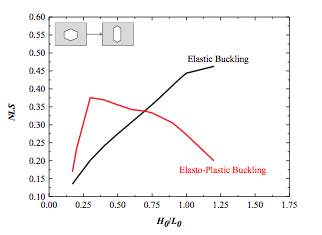Figure 8. Rectangular plate with transversal hexagonal hole

## 9.png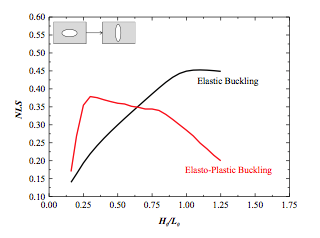Figure 9. Rectangular plate with elliptical hole

## 10.png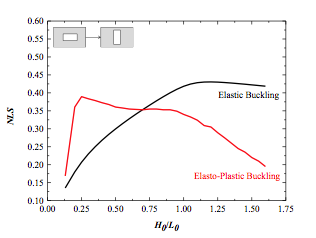Figure 10. Rectangular plate with rectangular hole

From Figs. 6, 7, 8, 9 and 10, in a general way it can be said that for small values of the ratio H0/L0 there is a predominance of elastic buckling and for larger values the dominance becomes the elasto-plastic buckling.

The transition between the elastic and elasto-plastic buckling has a specific point defined in terms of the DOF H0/L0 by values of 0.55, 0.55, 0.70, 0.64 and 0.70, respectively, for the diamond (Fig. 6), longitudinal hexagonal (Fig. 7), transversal hexagonal (Fig. 8), elliptical (Fig. 9) and rectangular (Fig. 10) hole.

Therefore, Fig. 11 shows the limit curves for the maximum NLS which each perforated plate can support without to suffer the buckling phenomenon.

One can note in Fig. 11 that the maximum global NLS for the plate with H/L = 0.5 was obtained with the rectangular cutout with (H0/L0)opt = 0.70 and reaching a (NLS)max value of 0.36. If this best case is compared with the lower maximum NLS obtained (diamond hole) an improvement of 37.25% was

achieved only due to the cutout type. However, the rectangular perforation is not the best global hole type, because for the H0/L0 range between 0.25 and 0.66 the superior plate performance was observed for the longitudinal hexagonal perforation. In addition, for specific values of H0/L0 the elliptical and diamond hole also presented superior performances if compared with the rectangular cutout.

## 11.pngFigure 11. Elastic Buckling and elasto-plastic buckling of rectangular perforated plate

In Table 1, considering only the elasto-plastic buckling of the plates with H/L = 0.5, it is presented a comparison between the values of the optimal geometry and maximum NLS with the worst geometry and minimum NLS for each hole type. It is observed that improvements around 60.00%, 52.17%, 70.00%, 75.00% and 80.00% can be obtained, respectively for the diamond, hexagonal, elliptical and rectangular cutouts, if the adequate hole geometry is employed.

Table 1. Comparison between best and worst shape for each hole type for the plate with H/L = 0.5

 Hole Type (H0/L0)opt (NLS)max H0/L0 NLS NLS Difference % Diamond 0.51 0.32 0.80 0.20 60.00 Longitudinal Hexagonal 0.55 0.35 1.09 0.23 52.17 Transversal Hexagonal 0.70 0.34 1.20 0.20 70.00 Elliptical 0.64 0.35 1.25 0.20 75.00 Rectangular 0.70 0.36 1.60 0.20 80.00

Figures 12, 13, 14, 15 and 16 show the von Mises stress distribution in studied rectangular plates (H/L = 0.5) considering the cases presented in Table 1, for the diamond, longitudinal hexagonal, transversal hexagonal, elliptical and rectangular holes, respectively.

## 12.pngFigure 12. Stress distribution in plates with diamond hole: (a) optimal shape and (b) worst shape

S

## 13.pngFigure 13. Stress distribution in plates with longitudinal hexagonal hole: (a) optimal shape and (b) worst shape

## 14.png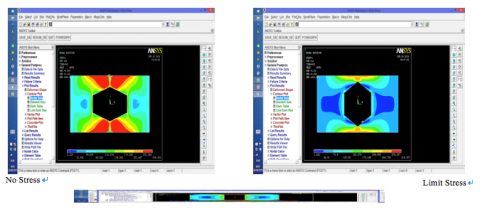Figure 14. Stress distribution in plates with transversal hexagonal hole: (a) optimal shape and (b) worst shape

## 15.pngFigure 15. Stress distribution in plates with elliptical hole: (a) optimal shape and (b) worst shape

## 16.png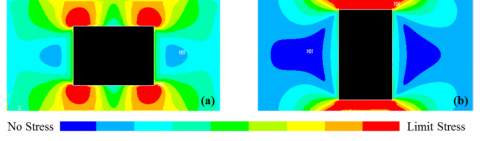Figure 16. Stress distribution in plates with rectangular hole: (a) optimal shape and (b) worst shape

It is possible to note in Figs. 12, 13, 14, 15 and 16 that the optimal cutout shapes promote a better distribution of the maximum stress, i.e., there are more regions submitted to the maximum stress in the optimal geometries, being this behavior in agreement with the Constructal principle of the optimal distribution of imperfections.

Now, the NLS variation is depicted as function of the DOF H0/L0 for the square plates (H/L = 1.0) with diamond (Fig. 17), longitudinal hexagonal (Fig. 18), transversal hexagonal (Fig. 19), elliptical (Fig. 20) and rectangular (Fig. 21) perforations.

## 17.png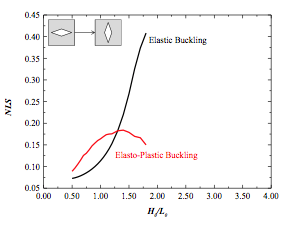Figure 17. Square plate with diamond hole

## 18.pngFigure 18. Square plate with longitudinal hexagonal hole.

## 19.pngFigure 19. Square plate with transversal hexagonal hole

## 20.png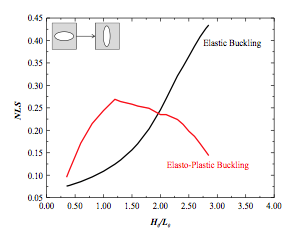Figure 20. Square plate with elliptical hole

## 21.pngFigure 21. Square plate with rectangular hole

The H0/L0 value that define the transition from elastic buckling, to the elasto-plastic buckling are 1.30, 1.68, 1.98, 1.94 and 2.23 for the plates with diamond (Fig. 17), longitudinal hexagonal (Fig. 18), transversal hexagonal (Fig. 19), elliptical (Fig. 20) and rectangular (Fig. 21) holes.

From Figs. 17, 18, 19, 20 and 21 the NLS limit curves to avoid the buckling occurrence can be defined adding the elastic behavior portion (on the left of the intersection point) and the elasto-plastic behavior portion (on the right of theintersection point) for each hole type, as can be seen in Fig. 22. As already observed for the plates with H/L = 0.5 (see Fig. 11), the rectangular hole conduct to the global superior performance with (H0/L0)opt = 2.23 and (NLS)max = 0.25. This geometry has a mechanical behavior approximately 64% better than the best case among the studied plates with diamond holes.

## 22.pngFigure 22. Elastic buckling and elasto-plastic buckling of square plate perforated

Again, in some specific regions the rectangular hole do not conducts to the superior performance, despite having reached the highest NLS level. Diamond (1.15 ≤ H0/L0 ≤ 1.40), hexagonal (1.40 ≤ H0/L0 ≤ 1.90), and elliptical (1.90 ≤ H0/L0 ≤ 2.15) perforations can be more efficient depending of the H0/L0 value.

In Table 2, considering only the elastic-plastic buckling behavior and for each hole type, the optimized shape and the maximum NLS value is confronted with the worst shape and the minimum NLS value. It is observed that, for the square plates the variation between maximum and minimum NLS values can generate performance improvements of 20.00%, 20.00%, 46.67%, 64.29% and 78.57%, respectively, for diamond, hexagonal, elliptical and rectangular hole types.

Table 2. Comparison between best and worst shape for each hole type for the plate H/L = 1.0.

 Hole Type (H0/L0)opt (NLS)max H0/L0 NLS NLS Difference % Diamond 1.30 0.18 1.80 0.15 20.00 Longitudinal Hexagonal 1.68 0.24 2.31 0.20 20.00 Transversal Hexagonal 1.98 0.22 2.76 0.15 46.67 Elliptical 1.94 0.23 2.89 0.14 64.29 Rectangular 2.25 0.25 3.65 0.14 78.57

For the cases showed in Table 2, the von Mises stress distribution are illustrated in Fig. 23 (diamond), Fig. 24 (longitudinal hexagonal), Fig. 25 (transversal hexagonal), Fig. 26 (elliptical), and Fig. 27 (rectangular).

## 23.pngFigure 23. Stress distribution plates with diamond hole: (a) optimal shape and (b) the worst shape.

## 24.png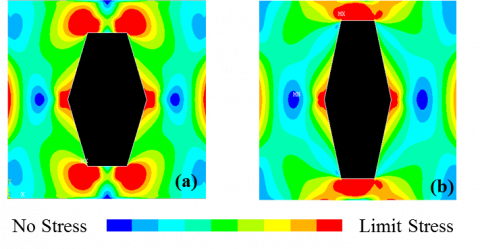Figure 24. Stress distribution plates with longitudinal hexagonal hole: (a) optimal shape and (b) the worst shape.

## 25.pngFigure 25. Stress distribution plates with transversal hexagonal hole: (a) optimal shape and (b) the worst shape.

## 26.pngFigure 26. Stress distribution plates with elliptical hole: (a) optimal shape and (b) the worst shape.

## 27.png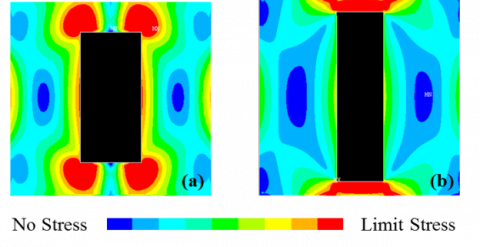Figure 27. Stress distribution plates with rectangular hole: (a) optimal shape and (b) the worst shape.

As noted in the plates with H/L = 0.5, the optimized geometries have more regions where the maximum NLS is reached, totaling a larger area submitted to the maximum stress. Therefore, the principle of optimal distribution of imperfections is respected for these plates, conducting to a superior performance.

6. Conclusions

The present work shows that the hole type and shape have a fundamental importance for the definition of the buckling behavior in perforated plates. The adequate hole type choice allow to obtain a superior performance, i.e., removing the same material amount of the plate is possible to improve its performance only by the correct hole type choice. In addition, for all studied hole types, its shape variation promoted by means the DOF H0/L0 is responsible to define if the buckling will be elastic (linear) or elasto-plastic (nonlinear). For lower values of H0/L0 an elastic buckling occur while an elasto-plastic buckling happens for higher values for the ratio H0/L0. Hence, there is an intersection point between the curves that define the elastic and elasto-plastic plate buckling. This point determines the transitions between these mechanical behaviors, usually also being where the level maximum of the stress is reached among all studied DOF H0/L0 for each hole type.

Another important observation is that there is no optimal global geometry. Depending of the H0/L0 value, a particular hole type presents the best performance. This is an important aspect if the cutout at the plate need to be done with a specific geometry.

For each hole type, considering only the elasto-plastic plate buckling behavior and comparing the best and worst geometries one can note that the decrease in the area between the hole and the upper and lower edges of the plate leads to the collapse of the structure. This fact can be explained by the decreasing of the plate resistant area. In addition, the best geometry always have a better stress distribution, in accordance with the Constructal principle of optimal distribution of imperfections, justifying its superior performances.

In general it can be said that the studied rectangular perforated plates (H/L = 0.5) and square perforated plates (H/L = 1.0) have a similar buckling behavior. However, the rectangular perforated plates can support a more elevated stress level when compared with the square perforated plates. As the total volume of the plate material is the same in both cases, if possible it is recommended to use the rectangular perforated plate.

Therefore, the Constructal Design method proved to be able to analyze the geometric configuration influence at the plate buckling problem, allowing to define for each studied hole type a limit stress curve as function of the hole shape variation which avoids the buckling phenomenon occurrence.

Acknowledgment

The authors acknowledge FURG and UFRGS by the support. D. Helbig thanks to CNPq by the doctoral scholarship. L. A. O. Rocha, L. A. Isoldi, and E. D. dos Santos thanks CNPq by research grant.

References

1. El-Sawy, K. M., Nazmy, A.S., & Martini, M.I., “Elasto-plastic buckling of perforated plates under uniaxial compression,” Thin-Walled Structures, vol. 42, 1083–1101, 2004. DOI: 10.1016/j.tws.2004.03.002.

2. Iyengar, N.G.R., Structural Stability of Columns and Plates, Ellis Horwood Limited, 1988.

3. Narayanan R., Chow F.Y., “Ultimate capacity of uniaxially compressed perforated plates,” Thin-Walled Structures, vol. 2, 241–64, 1984. DOI: 10.1016/0263-8231(84)90021-1.

4. Shakerley, T. M., Brown, C.J., “Elastic buckling of plates with eccentrically positioned rectangular perforations,” International Journal of Mechanical Science, vol. 38, 825–838, 1996. DOI: 10.1016/0020-7403(95)00107-7.

5. El-Sawy, K.M., Nazmy, A.S., “Effect of aspect ratio on the elastic buckling of uniaxially loaded plates with eccentric holes,” Thin-Walled Structures, vol. 39, 983–998, 2001. DOI: 10.1016/S0263-8231(01)00040-4.

6. El-Sawy, K.M., Martini, M.I., “Elastic stability of bi-axially loaded rectangular plates with a single circular hole,” Thin-Walled Structures, vol. 45, 122–133, 2007. DOI: 10.1016/j.tws.2006.11.002.

7. Moen, C.D., Schafer, B.W., “Elastic buckling of thin plates with holes in compression or bending,” Thin-Walled Structures, vol. 47, 1597–1607, 2009. DOI: 10.1016/j.tws.2009.05.001.

8. Rocha, L.A.O., Real, M.V., Correia, A.L.G., Vaz, J., dos Santos, E.D., & Isoldi L.A., “Geometric optimization based on the constructal design of perforated thin plates subject to buckling,” Computational Thermal Sciences, vol. 4, n. 2, 119–129, 2012. DOI: 10.1615/ComputThermalScien.2012005125.

9. Rocha, L.A.O., Isoldi L.A., Real, M.V., dos Santos, E.D., Correia, A.L.G., Lorenzini, G., Biserni, C., “Constructal design applied to the elastic buckling of thin plates with holes,” Central European Journal of Engineering, vol. 3, 475–483, 2013. DOI: 10.2478/s13531-013-0105-x.

10. Isoldi, L.A., Real, M.V., Correia, A.L.G., Vaz, J., dos Santos, E.D., & Rocha, L.A.O., “The flow of stresses: constructal design of perforated plates subjected to tension or buckling,” In Rocha, L.A.O., Lorente, S., and Bejan, A., eds., Constructal Law and the Unifying Principle of Design, 195–217, Springer-Verlag, 2013. DOI: 10.1007/978-1-4614-5049-8_12.

11. Narayanan R, Chow F.Y., “Ultimate capacity of uniaxially compressed perforated plates,” Thin-Walled Structures, vol. 2, 241–64, 1984. DOI: 10.1016/0263-8231(84)90021-1.

12. Azizan Z.G., Roberts T.M., “Buckling and elasto-plastic collapse of perforated plates,” Proceedings of the Michael R, Horne Conference on Instability and Plastic Collapse of Steel Structures, Manchester, 322–328, 1983.

13. Yettram, A.L., Brown, C.J., “The elastic stability of square perforated plates,” Computers and Structures, Vol. 21, No. 6, 1267-1272, 1985. DOI: 10.1016/0143-974X(87)90014-9.

14. Jwalamalini, R., Sundaravadivelu, R., Vendhan, C.P. and Ganapathy, C., “Stability of initially stressed square plates with square openings,” Marine Structures, Vol. 5, No. 1, 71-84, 1992. DOI: 10.1016/0951-8339(92)90034-M.

15. Madasamy, C.M., Kalyanaraman, V., “Analysis of plated structures with rectangular cutouts and internal supports using the spline finite strip method,” Computers and Structures, Vol. 52, No. 2, 277-286, 1994. DOI: 10.1016/0045-7949(94)90280-1.

16. Durban, D., Zuckerman, Z., “Elastoplastic buckling of rectangular plates in biaxial compression/tension,” International Journal of Mechanical Science, vol. 41, 751–65, 1999. DOI: 10.1016/S0020-7403(98)00055-1.

17. Shanmugam, N.E., Thevendran, V., & Tan Y.H., “Design formula for axially compressed perforated plates,” Thin-Walled Structures, vol. 34, 1–20, 1999. DOI: 10.1016/S0263-8231(98)00052-4.

18. Paik, J.K., Thayamballi, A.K. and Kim, B.J., “Advanced ultimate strength formulations for ship plating under combined biaxial compression/tension, edge shear, and lateral pressure loads,” Marine Technology, Vol. 38, No. 1, 9-25, 2001.

19. Toulios, M., Caridis, P.A., “The effect of aspect ratio on the elastoplastic response of stiffened plates loaded in uniaxial edge compression,” Computers and Structures, Vol. 80, No. 14-15, 1317-1328, 2002. DOI: 10.1016/S0045-7949(02)00080-9.

20. Bakker, M.C.M., Rosmanit, M., Hofmeyer, H., Post-Buckling Strength of Uniformly Compressed Plates, Stability and Ductility of Steel Structures, D. Camotim et al. (Eds.), Lisbon, Portugal, 2006.

21. Kumar et al., “Ultimate strength of square plate with rectangular opening under axial compression,” Journal of Naval Architecture and Marine Engineering, Vol. 4, 15-26, 2007. DOI: 10.3329/jname.v4i1.913.

22. Helbig, D., Real, M.V., Correia, A.L.G., Dos Santos, E. D., Isoldi, L.A., Rocha, L.A.O., “Constructal design of perforated steel plates subject to linear elastic and nonlinear elasto-plastic buckling,” XXXIV Iberian Latin American Congress on Computational Methods in Engineering (CILAMCE), 1–17, 2013.

23. Helbig, D., Rocha, L.A.O., Dos Santos, E.D., Real, M. V., Isoldi, L.A., Silva, C.C.C., “Numerical simulation and constructal design method applied to the study of the cutout shape influence in the mechanical behavior of perforated plates subjected to buckling,” XXXV Iberian Latin American Congress on Computational Methods in Engineering (CILAMCE), 2014.

24. Dawe, J.L. et al., “Inelastic buckling of steel plates,” Journal of Structural Engineering, Vol. 3(1), 1985. DOI: 10.1061/(ASCE)0733-9445(1985)111:1(95).

25. Raviprakash, A.V., “Investigations on the ultimate strength of axially compressed thin square plates with geometrical imperfections,” Thesis (Doctor of Philosophy in Mechanical Engineering) – Department of Mechanical Engineering, Pondicherry University, India, 2012.

26. Ventsel, E., Krauthammer, T., Thin Plates and Shells – Theory, Analysis, and Applications, Marcel Dekker, Inc., 2001. DOI: 10.1115/1.1483356.

27. Åkesson, B., Plate Buckling in Bridges and Other Structures, Taylor & Francis, 2007.

28. Yoo, C.H., Lee, S.C., Stability of Structures - Principles and Applications, Elsevier, 2011.

29. Vinson, J.R., Plate and Panel Structures of Isotropic, Composite and Piezoelectric Materials, Including Sandwich Construction, Springer, 2005. DOI: 10.1007/1-4020-3111-4.

30. Yamaguchi, E., Basic Theory of Plates and Elastic Stability, Chen Wai-Fah, 1999. DOI: 10.1201/9781439834350.ch1.

31. Moaveni, S., Theory and Application with ANSYS®, Prentice Hall, 1999.

32. Real, M. de V., Isoldi, L.A., “Finite Element Buckling Analysis of Uniaxially Loaded Plates with Holes,” IV Southern Conference on Computational Modeling (MCSUL), 69–73, 2010.

33. ANSYS®, User’s manual. Swanson Analysis System Inc., 2005.

34. Madenci, E., Guven, I., The Finite Element Method and Applications in Engineering Using ANSYS®, Springer, 2006. DOI: 10.1007/978-1-4899-7550-8.

35. Przemieniecki, J.S., Theory of Matrix Structural Analysis, Dover Publications, 1985.

36. Budiansky, B., “Notes on nonlinear shell theory,” Journal of Applied Mechanics, Vol. 35, Nº 2, pp. 393-401, 1968. DOI: 10.1115/1.3601208.

37. Avner, H.S., Introduction to Physical Metallurgy, Tata McGraw Hill Publishing Company, 2001.

38. Bejan, A., “Constructal-theory network of conducting paths for cooling a heat generating volume,” International Journal of Heat and Mass Transfer, vol. 40, 799–816, 1997. DOI: 10.1016/0017-9310(96)00175-5.

39. Bejan, A., Shape and Structure, from Engineering to Nature, Cambridge University Press, 2000. DOI: 10.3390/e3050293.

40. Bejan, A., Lorente, S., Design with Constructal Theory, Wiley, 2008. DOI: 10.1002/9780470432709.

41. Ghodoossi, L., Egrican, N., “Conductive cooling of triangular shaped electronics using constructal theory,” Energy Conversion and Management, vol. 93(8), 4922-4929, 2003. DOI: 10.1016/S0196-8904(03)00190-0.